Function Repository Resource:

# SymmetricDifference

The complement of the union and intersection of lists, with duplicates deleted

Contributed by: George Beck
 ResourceFunction["SymmetricDifference"][lists] gives the complement of the Union and Intersection of lists.

## Examples

### Basic Examples (2)

The common element 3 is removed from the Union of the sets:

 In:=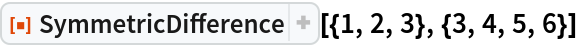Out=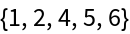Define three lists:

 In:=Here is their Union:

 In:=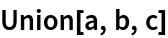Out=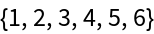Here is their Intersection:

 In:=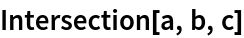Out=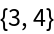Using those, you can define their symmetric difference:

 In:=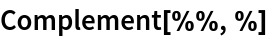Out=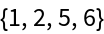SymmetricDifference gives the same result:

 In:=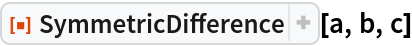Out=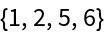### Possible Issues (3)

First define four regions:

 In:=The function RegionSymmetricDifference is like the resource function MultisetSymmetricDifference, not SymmetricDifference:

 In:=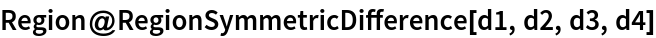Out=This construction is like SymmetricDifference:

 In:=Out=George Beck

## Version History

• 1.0.0 – 22 May 2019